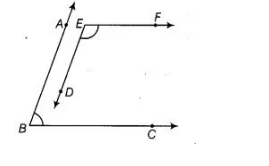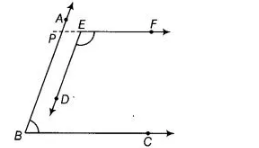# In the figure, BA || ED and BC || EF.`
Question:

In the figure, BA || ED and BC || EF. Show that ∠ABC + ∠DEF = 180°.Solution:

Given BA || ED and BC || EF

To show, ∠ABC + ∠DEF = 180°

Construction Draw a ray PE opposite to ray EF.Proof in the figure, $B C \| E F$

$\therefore \angle E P B+\angle P B C=180^{\circ}$ [sum of cointerior angles is $180^{\circ}$ ] ...(i)

Now, $A B \| E D$ and $P E$ is a transversal line.

$\therefore \quad \angle E P B=\angle D E F \quad$ [corresponding angles] ...(ii)

From Egs. (i) and (ii), $\quad \angle D E F+\angle P B C=180^{\circ}$

$\Rightarrow \quad \angle A B C+\angle D E F=180^{\circ}$ $[\because \angle P B C=\angle A B C]$